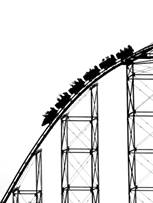Year 11
Year 11# Physics Units 1 & 2

## Unit 1: What ideas explain the physical world?

This unit of Physics explores the ideas that explain the physical world at various levels. They examine some of the fundamental ideas and models used by physicists in an attempt to understand and explain the world. Students use thermodynamic principles to investigate thermal energy, they apply thermal laws to investigate energy transfer and look at human impact on the use of energy and the environment. Students undertake regular experimental work in the laboratory starting with simple observations and measurements. The use of simple mathematical modelling, including calculations, is introduced to organise first-hand and second-hand data in order to make predictions and link concepts. Computer and graphics calculator programs are used to collect and analyse data and to present investigation findings. Students make and test predictions, identify discrete and continuous variables, select relevant independent variables and recognise controlled variables.

## AREAS OF STUDY:

• How can thermal effects be explained?
• How do electric circuits work?
• What is matter and how is it formed?## Unit 2: What do experiments reveal about the physical world?

This unit focuses on students exploring the power of experiments in developing models and theories. Students generate questions and investigate the ways in which forces are involved in both moving objects and keeping them stationary. The use of both independent and continuous variables is incorporated into experiments and students take increasing responsibility for the design of investigations. Students continue to undertake extensive and regular experimental work in the laboratory.

## AREAS OF STUDY:

• How can motion be described and explained?
• Selected Option based on Physical World
• Practical Investigation

## Unit 3: How do fields explain motion and electricity?

In this unit students explore the importance of energy in explaining and describing the physical world. They examine the production of electricity and its delivery to homes. Students consider why objects move when they are not apparently in contact with other objects. Applications of concepts related to fields include the transmission of electricity over large distances and the design and operation of particle accelerators. They explore the interactions, effects and applications of gravitational, electric and magnetic fields. Students use Newton’s laws to investigate motion in one and two dimensions, and are introduced to Einstein’s theories to explain the motion of very fast objects. They consider how developing technologies can challenge existing explanations of the physical world, requiring a review of conceptual models and theories. Students design and undertake investigations involving at least two continuous independent variables.

## AREAS OF STUDY:

• How do things move without contact?
• How are fields used to move electrical energy?
• How fast can things go?

## Unit 4:

In this unit, students explore the use of wave and particle theories to model the properties of light and matter. They examine how the concept of the wave is used to explain the nature of light and explore its limitations in describing light behaviour. Students further investigate light by using a particle model to explain its behaviour. A wave model is also used to explain the behaviour of matter which enables students to consider the relationship between light and matter. Students learn to think beyond the concepts experienced in everyday life to study the physical world from a new perspective. Students design and undertake investigations involving at least two continuous independent variables.

## AREAS OF STUDY:

• How can waves explain the behaviour of light?
• How are light and matter similar?
• Practical Investigation